TitleCollege Algebra
Answer/Discussion to Practice Problems
Tutorial 31: Graphs of Functions, Part I:

Graping Functions by Plotting PointsAnswer/Discussion to 1a; x = -3, -2, -1, 0, 1, 2, 3

 Step 1:  Find at least four ordered pair solutions.

 I'm going to use a chart to organize my information.  A chart keeps track of the x values that you are using and the corresponding y value found when you used a particular x value. We will use the seven values of x that were given to find corresponding functional values to give us seven ordered pair solutions. Keep in mind that the functional value that we find correlates to the second or y value of our ordered pair.

 x(x, y) -3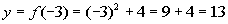(-3, 13) -2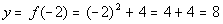(-2, 8) -1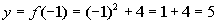(-1, 5) 0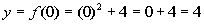(0, 4) 1(1, 5) 2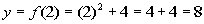(2, 8) 3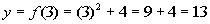(3, 13)

 Step 2:  Plot the points found in step 1.Step 3:  Draw the graph.

 This function is a quadratic function, so its graph will be a parabola.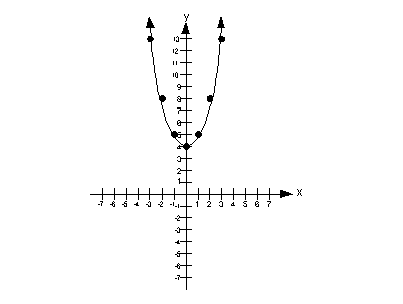Domain We need to find the set of all input values.  In terms of ordered pairs, that correlates with the first component of each one.  In terms of this two dimensional graph, that corresponds with the x values  (horizontal axis).  Since that is the case, we need to look to the left and right and see if there are any end points.  In this case, note how the curve has arrows at both ends, that means it would go on and on forever to the right and to the left.  This means that the domain is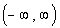.

 Range We need to find the set of all output values.  In terms of ordered pairs, that correlates with the second component of each one. In terms of this two dimensional graph, that corresponds with the y values (vertical axis).  Since that is the case, we need to look up and down and see if there are any end points.  In this case,  note how the curve has a low endpoint of y = 4 and it has arrows at both ends going up, that means it would go up on and on forever.  This means that the range is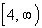.Answer/Discussion to 1b;  x = -3, -2, 1, 6

 Step 1:  Find at least four ordered pair solutions.

 I'm going to use a chart to organize my information.  A chart keeps track of the x values that you are using and the corresponding y value found when you used a particular x value. We will use the four values of x that were given to find corresponding functional values to give us four ordered pair solutions. Keep in mind that the functional value that we find correlates to the second or y value of our ordered pair.

 x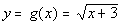(x, y) -3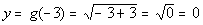(-3, 0) -2(-2, 1) 1(1, 2) 6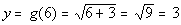(6, 3)

 Step 2:  Plot the points found in step 1.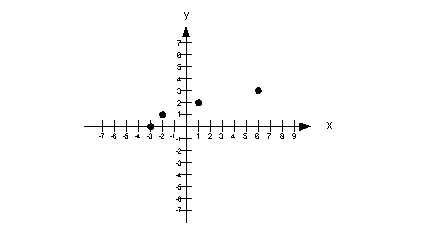Step 3:  Draw the graph.

 This function is a square root function, so its graph will be a curve.Domain We need to find the set of all input values.  In terms of ordered pairs, that correlates with the first component of each one.  In terms of this two dimensional graph, that corresponds with the x values  (horizontal axis).  Since that is the case, we need to look to the left and right and see if there are any end points.  In this case, note how there is a left endpoint at x = -3.  Also note that the curve has an arrow going to the right,  that means it would go on and on forever to the right.  This means that the domain is.

 Range We need to find the set of all output values.  In terms of ordered pairs, that correlates with the second component of each one. In terms of this two dimensional graph, that corresponds with the y  values (vertical axis).  Since that is the case, we need to look up and down and see if there are any end points.  In this case,  note how the curve has a low endpoint of y = 3 and it has an arrow  going up, that means it would go on and on forever up.  This means that the range is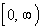.Answer/Discussion to 1c; x = -3, -2, -1, 0, 1, 2, 3

 Step 1:  Find at least four ordered pair solutions.

 I'm going to use a chart to organize my information.  A chart keeps track of the x values that you are using and the corresponding y value found when you used a particular x value. We will use the seven values of x that were given to find corresponding functional values to give us seven ordered pair solutions. Keep in mind that the functional value that we find correlates to the second or y value of our ordered pair.

 x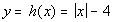(x, y) -3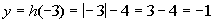(-3, -1) -2(-2, -2) -1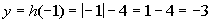(-1, -3) 0(0, - 4) 1(1, -3) 2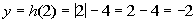(2, -2) 3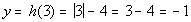(3, -1)

 Step 2:  Plot the points found in step 1.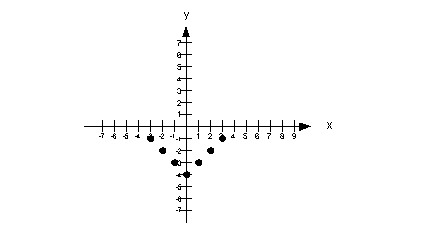Step 3:  Draw the graph.

 This function is an absolute value function, so its graph will be a v shape.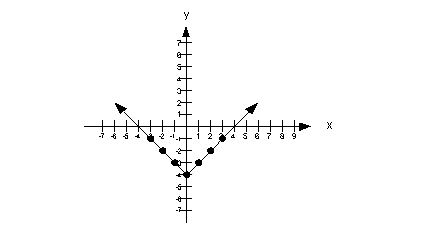Domain We need to find the set of all input values.  In terms of ordered pairs, that correlates with the first component of each one.  In terms of this two dimensional graph, that corresponds with the x values  (horizontal axis).  Since that is the case, we need to look to the left and right and see if there are any end points.  In this case, note how there are arrows at both ends, that means it would go on and on forever to the right and to the left.  This means that the domain is.

 Range We need to find the set of all output values.  In terms of ordered pairs, that correlates with the second component of each one. In terms of this two dimensional graph, that corresponds with the y  values (vertical axis).  Since that is the case, we need to look up and down and see if there are any end points.  In this case,  note how the graph has a low endpoint of y = -4 and it has an arrows that go up, that means it would go up on and on forever.  This means that the range is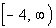.Answer/Discussion to 1d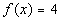;  x = -3, -2, -1, 0, 1, 2, 3

 Step 1:  Find at least four ordered pair solutions.

 I'm going to use a chart to organize my information.  A chart keeps track of the x values that you are using and the corresponding y value found when you used a particular x value. We will use the seven values of x that were given to find corresponding functional values to give us seven ordered pair solutions. Keep in mind that the functional value that we find correlates to the second or y value of our ordered pair.

 x(x, y) -3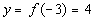(-3, 4) -2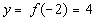(-2, 4) -1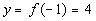(-1, 4) 0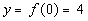(0, 4) 1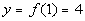(1, 4) 2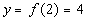(2, 4) 3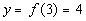(3, 4)

 Step 2:  Plot the points found in step 1.Step 3:  Draw the graph.

 This function is a constant function, so its graph will be a horizontal line.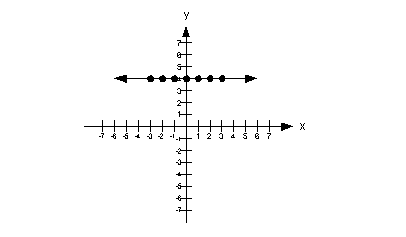Domain We need to find the set of all input values.  In terms of ordered pairs, that correlates with the first component of each one.  In terms of this two dimensional graph, that corresponds with the x values  (horizontal axis).  Since that is the case, we need to look to the left and right and see if there are any end points.  In this case, note how the curve has arrows at both ends, that means it would go on and on forever to the right and to the left.  This means that the domain is.

 Range We need to find the set of all output values.  In terms of ordered pairs, that correlates with the second component of each one. In terms of this two dimensional graph, that corresponds with the y  values (vertical axis).  Since that is the case, we need to look up and down and see if there are any end points.  In this case,  note how the line does not go up or down and that all the values of y are 4.  This means that the range is {y | y = 4}.Answer/Discussion to 1e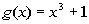; x = -2, -1, 0, 1, 2

 Step 1:  Find at least four ordered pair solutions.

 I'm going to use a chart to organize my information.  A chart keeps track of the x values that you are using and the corresponding y value found when you used a particular x value. We will use the five values of x that were given to find corresponding functional values to give us five ordered pair solutions. Keep in mind that the functional value that we find correlates to the second or y value of our ordered pair.

 x(x, y) -2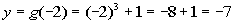(-2, -7) -1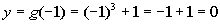(-1, 0) 0(0, 1) 1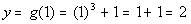(1, 2) 2(2, 9)

 Step 2:  Plot the points found in step 1.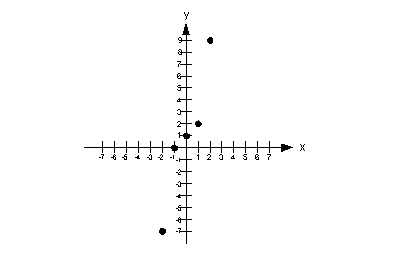Step 3:  Draw the graph.

 This function is a cubic function, so its graph will be a curve.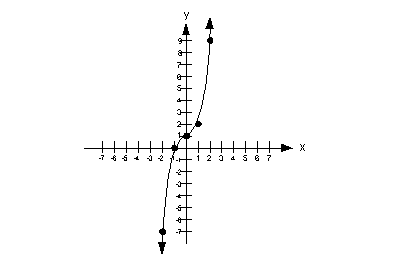Domain We need to find the set of all input values.  In terms of ordered pairs, that correlates with the first component of each one.  In terms of this two dimensional graph, that corresponds with the x values  (horizontal axis).  Since that is the case, we need to look to the left and right and see if there are any end points.  In this case, note how the curve has arrows at both ends, that means it would go on and on forever to the right and to the left.  This means that the domain is.

 Range We need to find the set of all output values.  In terms of ordered pairs, that correlates with the second component of each one. In terms of this two dimensional graph, that corresponds with the y  values (vertical axis).  Since that is the case, we need to look up and down and see if there are any end points. In this case, note how the curve has arrows at both ends, that means it would go on and on forever up and down. This means that the range is.

Last revised on April 7, 2010 by Kim Seward.
All contents copyright (C) 2002 - 2010, WTAMU and Kim Seward. All rights reserved.